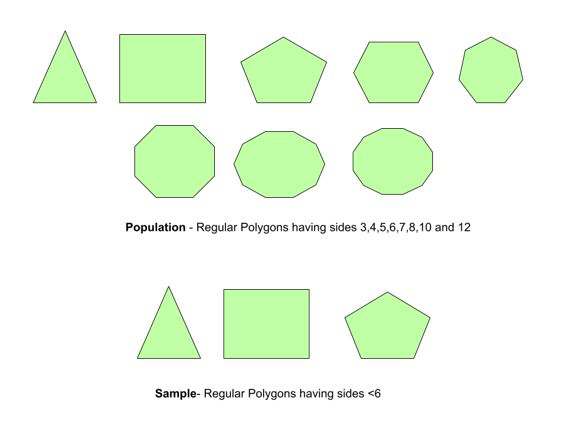Open in App
Not now

# Standard Deviation Formula

• Last Updated : 10 Jul, 2022

In statistics, the measure of the variation of values is known as Standard Deviation (SD). A low value of SD implies that the given set of values is spread over a small range, whereas a large value of SD means that the set of values is spread out over a large range. SD is often represented by the greek alphabet sigma σ  for population standard deviation or by the letter s for sample standard deviation. Now we shall see what is the difference between population and sample and the difference between their standard deviations. The population is defined as the entire group you want to study on. For Example All football players in Liverpool FC, All countries in the world, and all smartphones in the world. A sample is defined as a subgroup of a population under a specific condition. For Example All forward players in Liverpool FC, All countries that have an existent monarchy, All smartphones made by Apple

Population Vs SampleNote: The sample size is usually less than the population size but in some rare cases it can be the same. Now that we know the difference between population and sample let’s look at their standard deviations.

### Population Standard Deviation formula

σ =Here σ = Population Standard Deviation

xi = ith observation

μ = mean of N observation

N = number of observations.

If xi has different probabilities we use the formula,Where pi = probability of x

The above formulas are used when the value of x is finite. If we are given a continuous range of x we use the following,Here p(x) = Probability density function

E(x) = mean or expected value

### Sample Standard Deviation

The formula for sample SD is very similar to the population SD with minor changes,Here= mean of the sample

N = number of sample

Where does the N-1 come from?

The values in the sample will naturally be closer to the sample meanthan to the population mean μ. Thus to compensate for this, so that the SD is not underestimated, we use N – 1 instead of N in the denominator. If one wants to further read on it, feel free to google Unbiased estimation of standard deviation. It’ll give you the reason why we use N – 1 and not N – 2, etc.

Standard Deviation of Grouped Data

Now we shall see what to do if you’ve been given grouped data along with their frequency of occurrence. In Discrete Frequency Distribution, One value of X corresponds to one frequency. It will become clear from the sample problems below,

### Sample Problems

Problem 1: Find the Standard Deviation of X = {2, 2, 3, 4, 6, 7}.

Solution:

N = 6

x = {2, 2, 3, 4, 6, 7}

μ == (2 + 2 + 3 + 4 + 6 + 7)/ 6

= 4= (2 – 4)2 + (2 – 4)2 + (3 – 4)2 + (4 – 4)2 + (6 – 4)2 + (7 – 4)2

= 22σ = √(22/6)

= 1.914

Problem 2: Find the Standard deviation of X = {0, 1, 2, 3, 4} where the probability of each value is given by p(x) = {0.1, 0.3, 0.1, 0.2, 0.3}.

Solution:

μ = ∑ p(x) × x

= 2.3Problem 3: Find the standard deviation of X if the pdf of X is given by the function p(x) = (1 – x) for x ∈ [0, 1].

Solution:Problem 4: There were 30 students in the class. The weight of 5 is given as follows – 50Kg,35Kg, 80Kg, 45Kg, and 55Kg. Find the sample standard deviation.

Solution:Problem 5: Find the sample SD of the following data – {5, 6, 10, 12, 13}

Solution:Problem 6: Find the Standard Deviation of the following data,

Solution:Problem 7: Find the Standard Deviation of the following data table

Solution:My Personal Notes arrow_drop_up
Related Articles